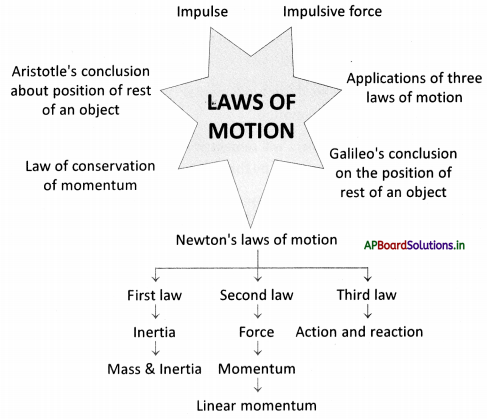# AP Board 9th Class Physical Science Notes Chapter 2 Laws of Motion

Students can go through AP State Board 9th Class Physical Science Notes Chapter 2 Laws of Motion to understand and remember the concept easily.

## AP State Board Syllabus 9th Class Physical Science Notes Chapter 2 Laws of Motion

→ Aristotle concluded that the natural state of an object is to be at rest.

→ According to Galileo, an object in motion will remain in the same motion as long as no external force is applied to it.

→ Sir Isaac Newton proposed his three fundamental laws which explain the connection between force and a change in motion.

→ The first law of Motion: Every object will remain at rest or in a state of uniform motion unless compelled to change its state by the action of a net force.

→ The tendency of matter that resists changes in its state of motion or rest is known as inertia.

→ As the mass is more, inertia is more.

→ Mass is a property of an object that specifies how much inertia the object has. SI unit of mass is the kilogram (kg).

→ The second law of Motion: The rate of change of momentum of a body is directly proportional to the net force acting on it and it takes place in the direction of the net force.

→ The linear momentum of a body is the product of its mass and velocity.
Momentum p = mass (m) × velocity (v)
SI unit of momentum is Kg-m /sec or Ns.

→ The net force Fnet = ma. SI unit of force is Kg-m/sec2 or ‘Newton’.

→ One ‘Newton’ is the force which when acting on a body of mass 1 kg, produces an acceleration of lm/sec2.
1 Newton = 1 kg × 1 ms-2→ Third Law of Motion: If an object exerts a force on the other object, the second object also exerts a force on the first one which is equal in magnitude but opposite in direction.

→ There exists no single isolated force, according to Newton’s third law of motion.

→ Flying a bird, swimming of a fish, launching a rocket, etc. are examples of Newton’s third law of motion.

→ The Law of conservation of momentum states in the absence of a net external force on the system, the momentum of the system remains unchanged.

→ A system is said to be isolated when the net external force acting on it is zero.

→ In an isolated system, the total momentum is conserved.

→ Laws of motion: Sir Isaac Newton proposed his fundamental laws which explain the connection between force and a change in motion. These three laws are popularly called Newton’s laws of motion.

→ Inertia: Inertia is a property of matter that resists changes in its state of motion or rest. It depends on the mass of the object.

→ Mass: The quantity of matter present in a body is known as its mass.

→ Linear momentum: Momentum of a body ¡s the product of its mass and velocity.
Momentum p = m × v

→ Newton used the word “mass in motion” to represent the meaning of momentum.

→ Conservation of momentum: Law of conservation of momentum states in the absence of net external force on the system. The momentum of the system remains unchanged.

→ Impulse: The product of net force and interaction time is called the impulse of the net force. It is equivalent to the change in momentum that an object experiences during an interaction.→ Impulsive force: Forces exerted over a limited time are called impulsive forces.→ Galileo Galilei:
Galileo Galilei was born on 15 February 1564 in Pisa, Italy. Galileo has been called the “father of modern science”.
In 1589, in his series of essays, he presented his theories about falling objects using an inclined plane to slow down the rate of descent.

Galileo was also a remarkable craftsman. He developed a series of telescopes whose optical performance was much better than that of Galileo Galilei’s other telescopes available during those days. Around 1640, he designed the first pendulum clock. In his book ‘StarryMessenger’ on his astronomical discoveries, Galileo claimed to have seen mountains on the moon, the MilkyWaymade up of tiny stars, and four small bodies orbiting Jupiter. In his books

‘Discourse on Floating Bodies’and ‘Letters on the sunspots, he disclosed his observations of sunspots. : Using his own telescopes and through his observations on Saturn and Venus, Galileo ‘ argued that lithe planets must orbit the Sun and not the earth, contrary to what was ‘ believed at that time.